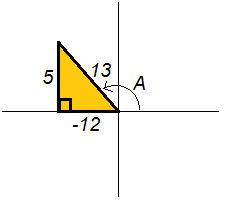# Formative Assessment Review

## Objective

SWBAT use trigonometric identities to solve a variety of problems.

#### Big Idea

Get students ready for their quiz and give them tools to check their work.

## Check Homework

20 minutes

Yesterday’s homework included questions that involved the sum and difference and double angle formulas. My plan is to give students the answers to the assignment and have them check their work. When I give this assignment, my students usually have the most trouble with missing negatives in Question #1 and the conceptual meaning of Question #6.

To address the mistakes with Question #1, give students the following strategies to make sure that they are getting the questions correct and not making procedural errors.

First Strategy: Make sure that students are drawing a diagram for every angle. For example, angle A is in quadrant II and its sine ratio is 5/13. Drawing a diagram like the one below will give place each angle in the correct quadrant and will give students a visual check as to whether or not each trig ratio should be positive or negative.Second Strategy: As discussed yesterday, students can check each of these values using the STORE function on their calculator. To get angle A, students can type in sin-1(5/12) to get an angle measure. They may notice that the angle is in quadrant I; have a quick discussion about why this is. Then see if they can find the exact angle in quadrant II that has the same sine value. Once they get that value without round at all, they can store this in their calculator as A. They can do the same thing for angle B. Once both angle measures are stored, students can type in tan(A + B), for example, to see if they get the same answer by using the calculator and using the formulas.

For question #6, students are to write cos(2arcsin x) as an algebraic expression. The most challenging part for students is recognizing that arcsin x is an angle measure, so I often rewrite it as cos(2A). Since we know that sin A = x/1, then the problem becomes much less abstract. Then, my students can use the double angle formula and can draw a sketch of the triangle to find the missing side length.

## Quiz Review

30 minutes

For this unit, more so than any other this year, students need an adequate amount of practice in order to master the concepts. This quiz review gives them practice with the formulas that we have learned so far. I will make a judgment call as to whether or not you want them to know these formulas or if they can use a formula sheet. In my experience, students can recall these formulas if they get enough practice, but it is just as important to know when to use them even if they have the formulas right in front of them.

Question #6 is a little different than the other problems and I discuss it in the video below.